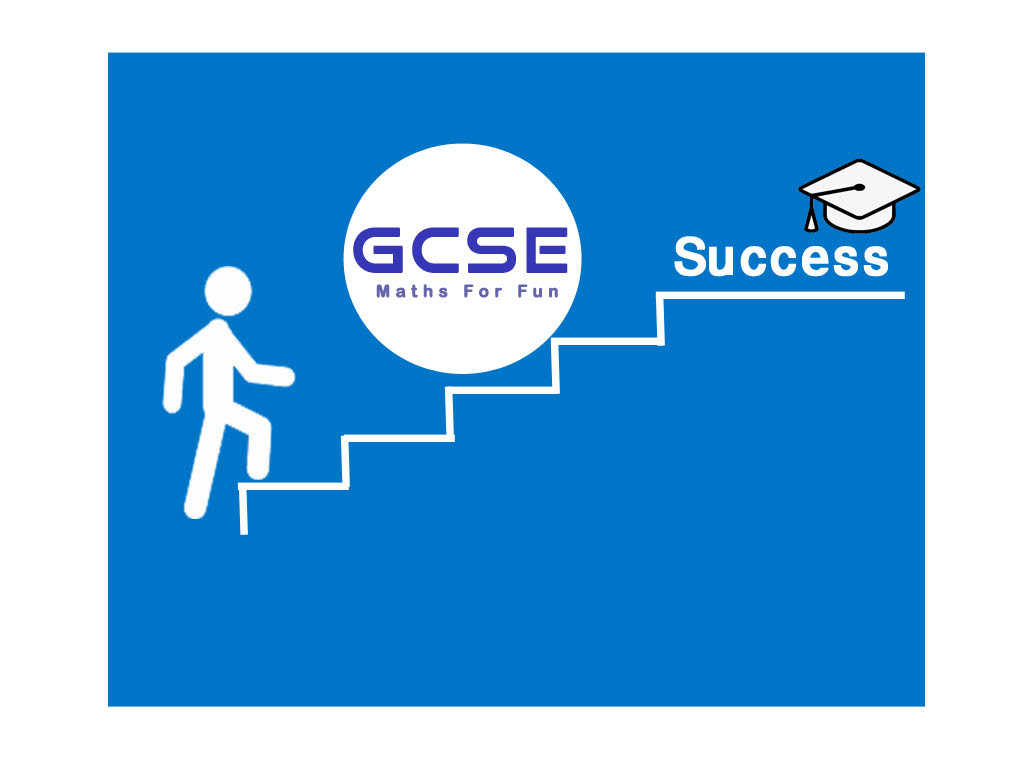Top

#### Basic Maths

Basic math is nothing but the simple or basic concept related with mathematics. Generally, counting, addition, subtraction, multiplication and division are called the basic math operation.

#### Algebra

The purpose of Algebra is to make it easy to state a mathematical relationship and its equation by using letters of the alphabet or other symbols to represent entities as a form of shorthand.

#### Geometric

Geometry. In its simplest form, geometry is the mathematical study of shapes and space. three-dimensional shapes with depth, such as cubes and spheres.

#### Trigonometric

There are three basic functions in trigonometry, each of which is one side of a right-angled triangle divided by another. You may find it helpful to remember Sine, Cosine and Tangent.

#### Calculus

There is a set of rules (product rule, quotient rule, chain rule) for manipulating and computing derivatives. There is also a set of rules (integration by parts, trig substitution, etc.)

#### Pratice Tests

most effective study techniques, study says. According to the study in Psychological Science in the Public Interest, students and teachers often use study techniques that are very effective

## Welcome to GCSEGcsemaths4fun.co.uk is a breakthrough in the world of educational websites. We use ground-breaking technology to help you achieve the best result you can in your Maths GCSE.